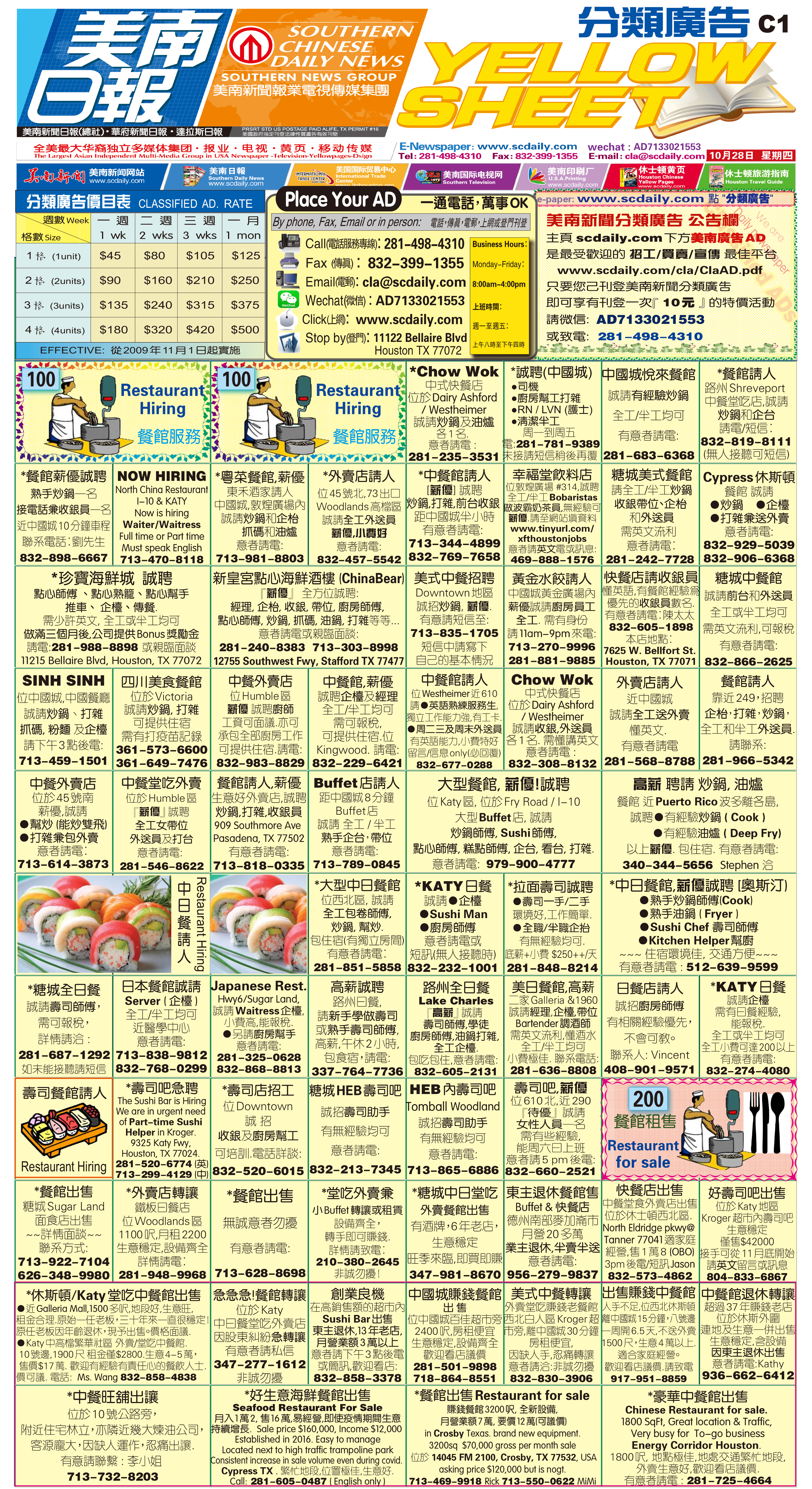211107 Epaper

 A Section B SectionC Section D Section211106 Epaper

 A Section B SectionC Section D Section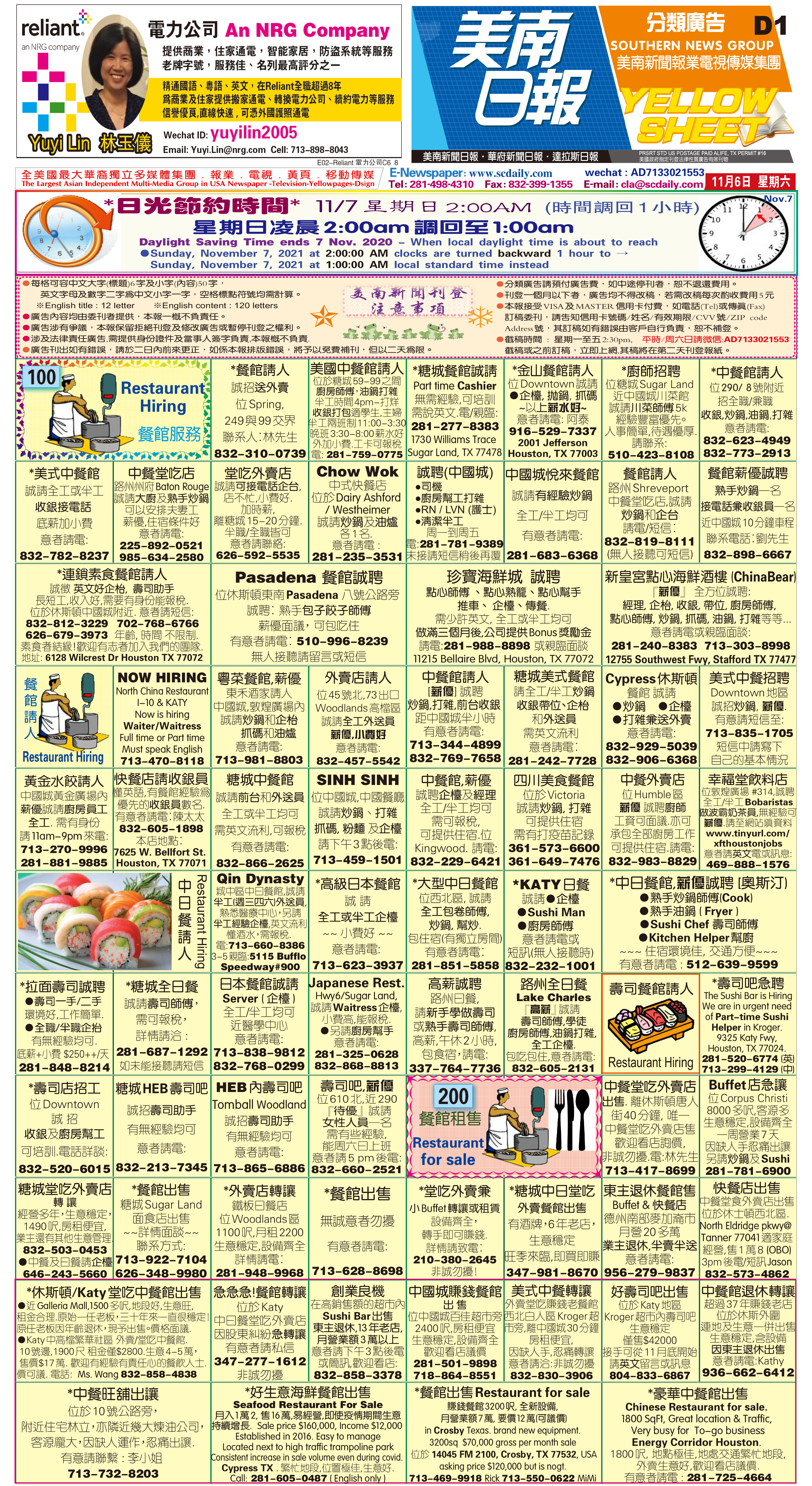211105 Epaper

 A Section B SectionC Section D Section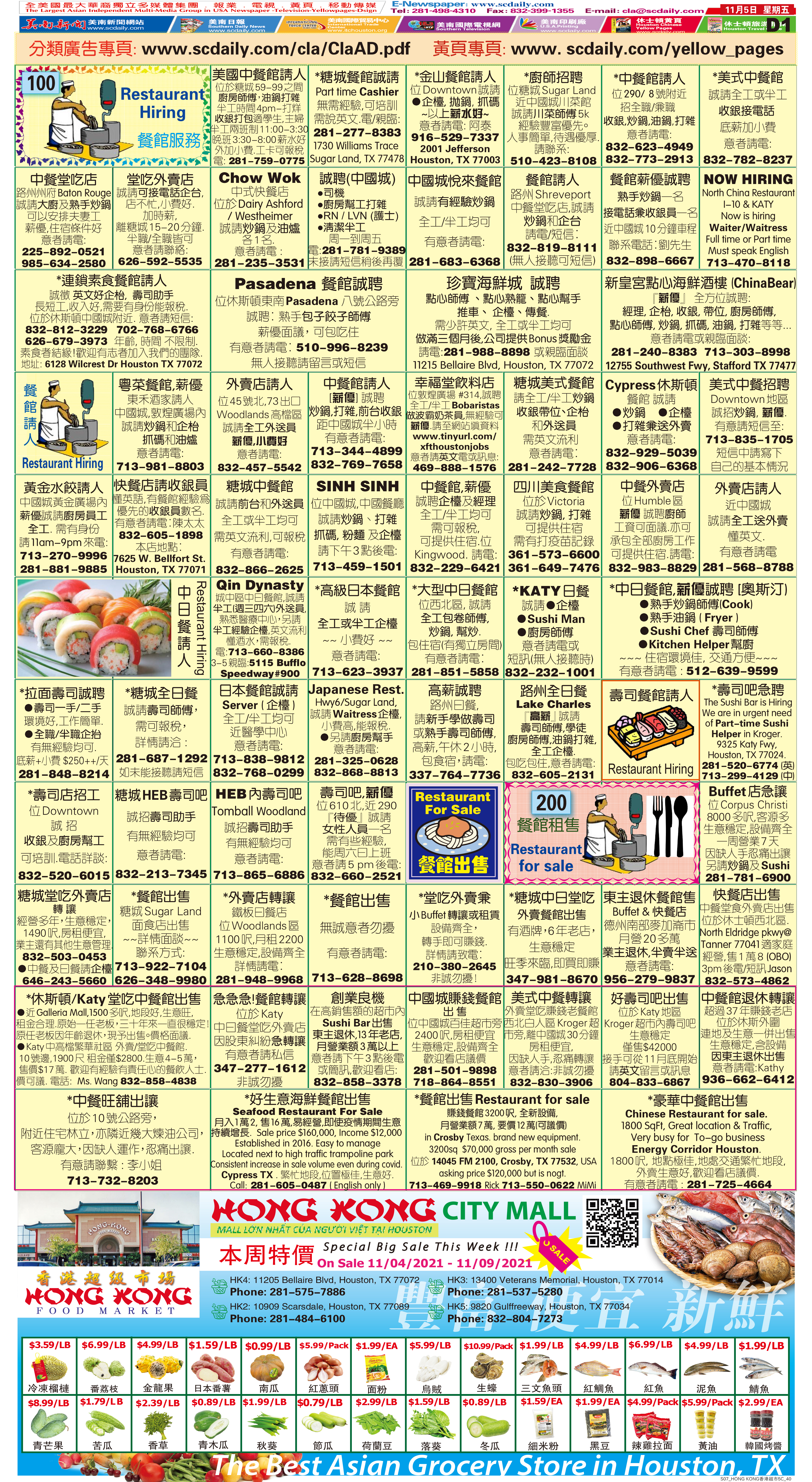211104 Epaper

 A Section B SectionC Section D Section211103 Epaper

 A Section B SectionC Section D Section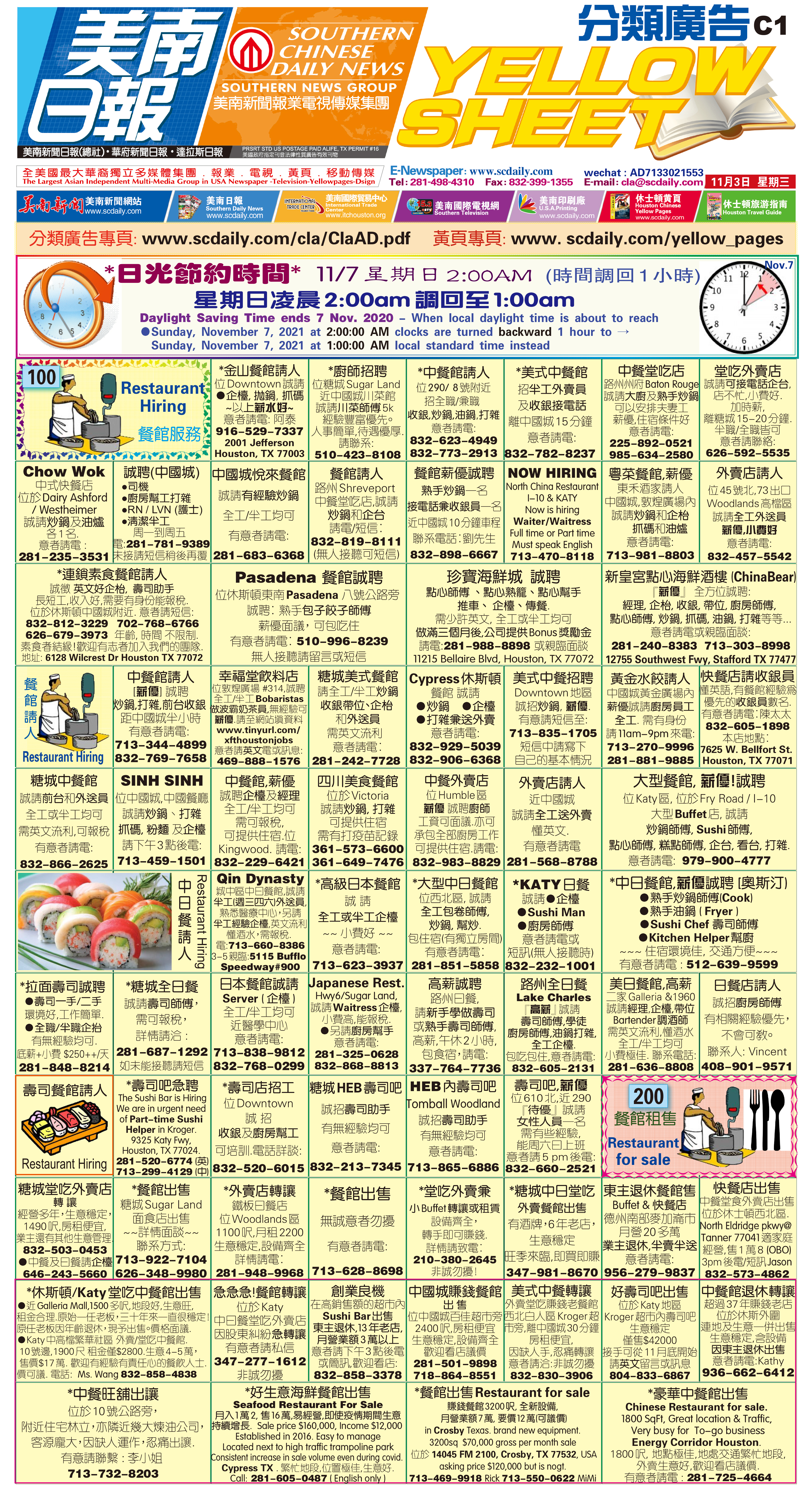211102 Epaper

 A Section B SectionC Section D Section211101 Epaper

 A Section B SectionC Section D Section211031 Epaper

 A Section B SectionC Section D Section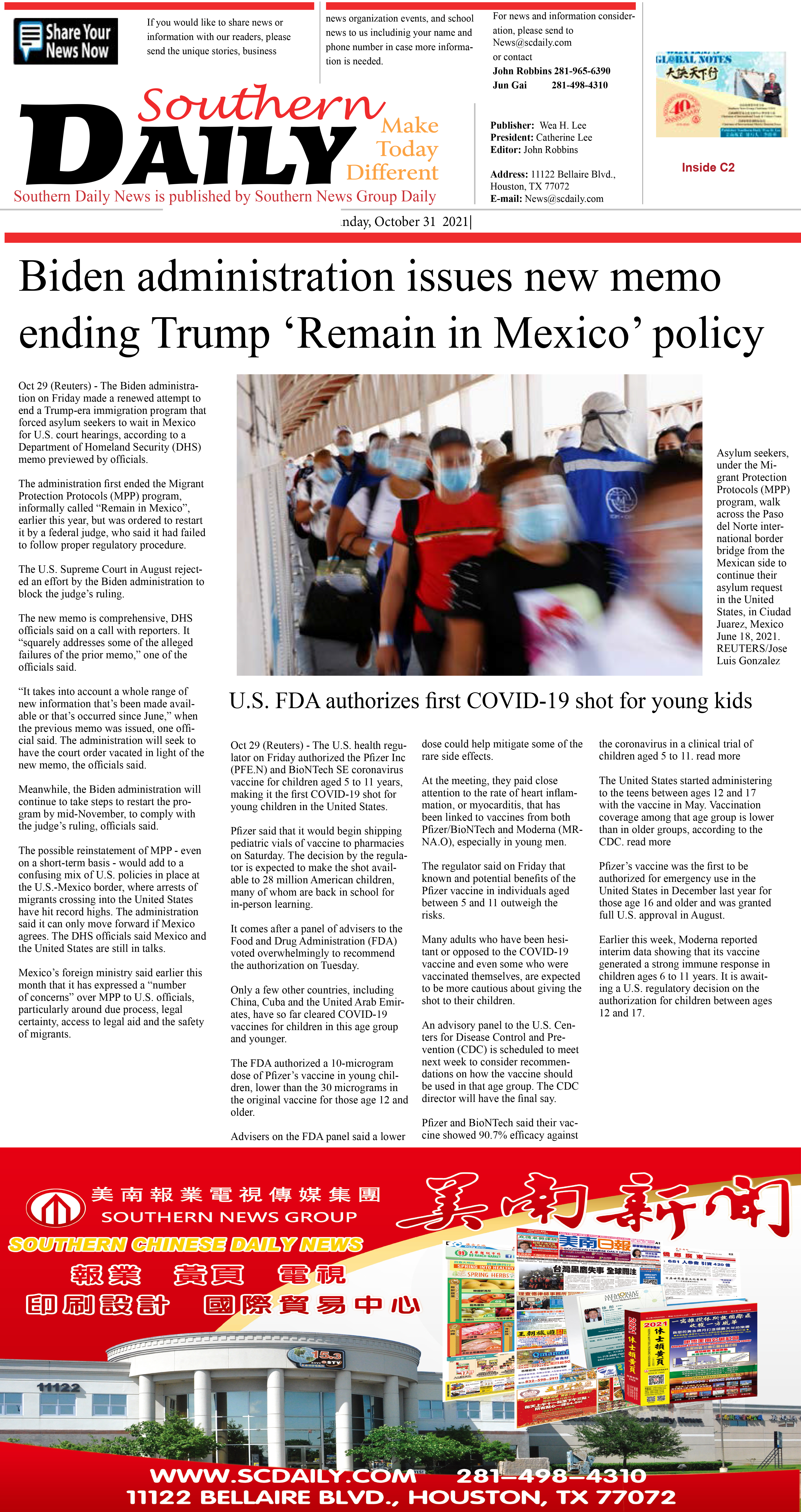211029 Epaper

 A Section B Section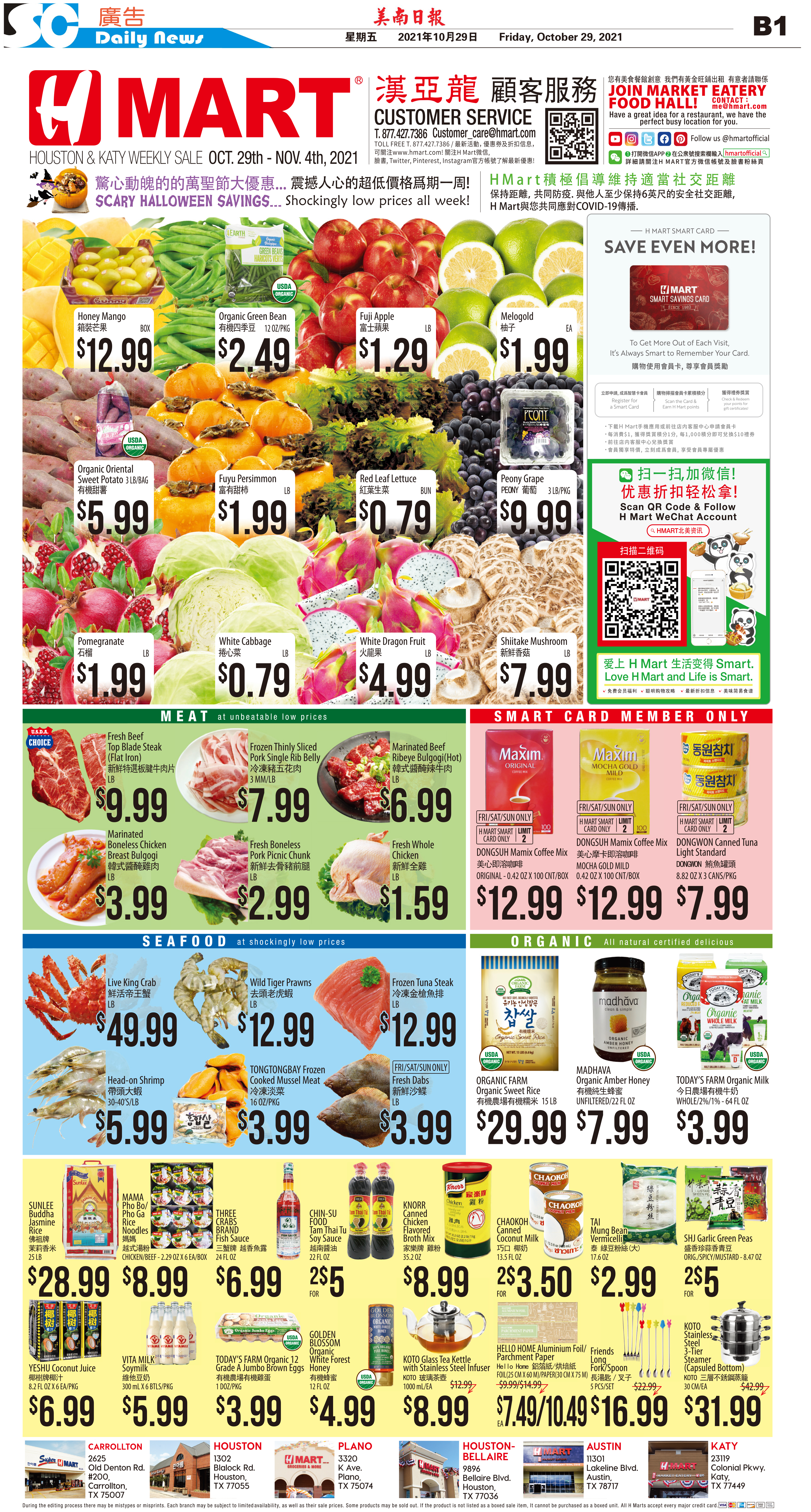C Section D Section211030 Epaper

 A Section B SectionC Section D Section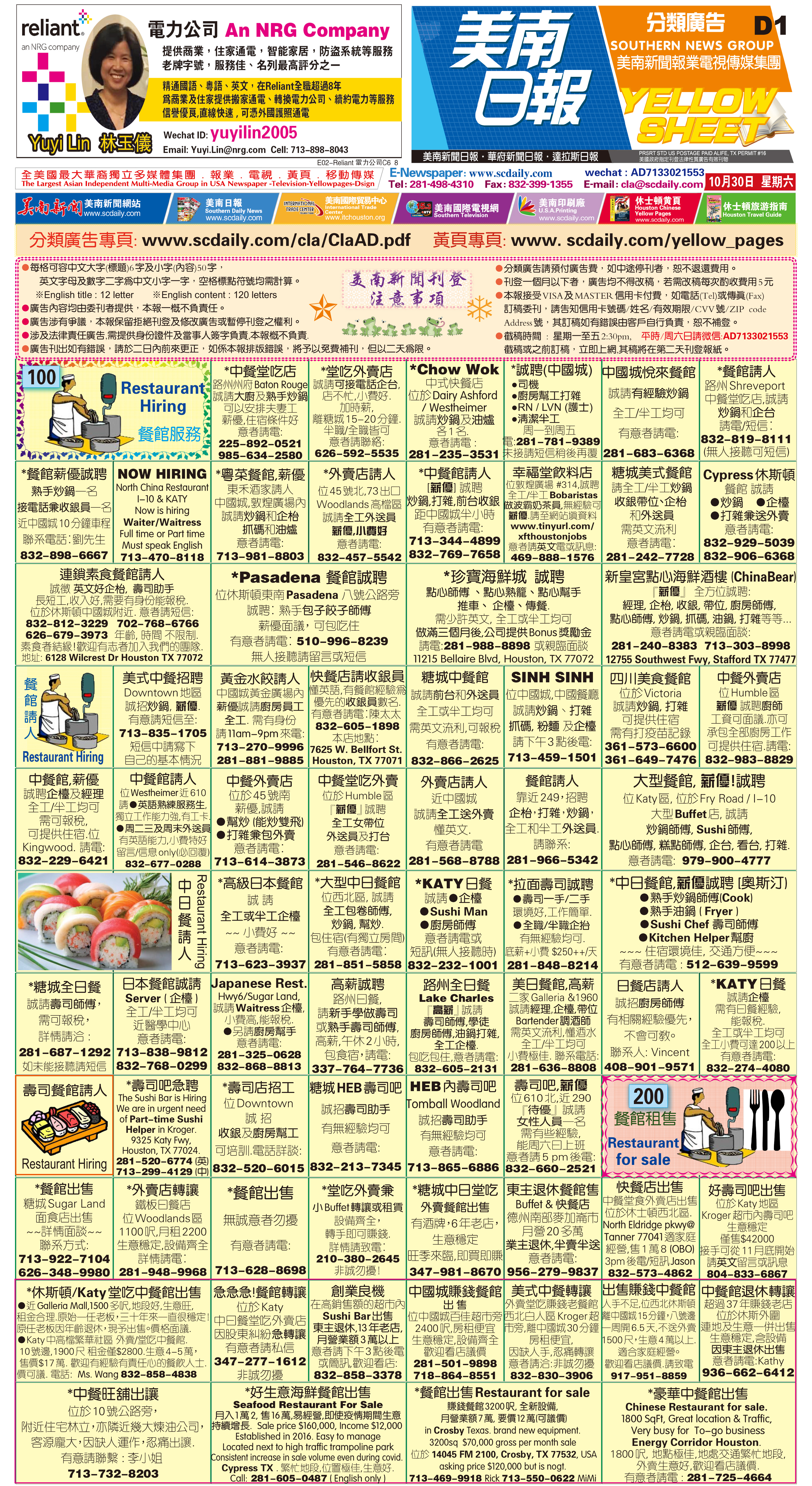211028 Epaper

 A Section B SectionC Section D Section